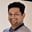Related Tags

factorial
java
communitycreator

# How to calculate factorial in JavaTarun Telang

The factorial of a non-negative number $n$ (denoted by $n!$) is the product of all the positive integers less than or equal to that number $n$.

Mathematically, it can be represented by the expression:

$n! = n \times (n-1) \times (n-2) \times ... \times 2 \times 1$

Example: The factorial of 6 is $6 \times 5 \times 4 \times 3 \times 2 \times 1 = 720$.

In order to calculate the factorial of a number in Java, use the following methods.

### Recursive approach

$factorial(n)$ = n × ($factorial(n-1)$)

The recursive program to calculate a factorial is as follows:

class Factorial {

public static int factorial(int n) {
if (n < 0)
throw new IllegalArgumentException("argument should be > 0");

return n == 0 ? 1 : n * factorial(n-1);
}
public static void main( String args[] ) {
System.out.println("0! -> " + factorial(0));
System.out.println("1! -> " + factorial(1));
System.out.println("3! -> " + factorial(3));
System.out.println("5! -> " + factorial(5));
System.out.println("10! -> " + factorial(10));
System.out.println("-1! -> " + factorial(-1));
}
}
Java code to calculate factorial of a number recursively

Note that the recursive algorithm factorial calculates $n!$ (where $n$ is a positive integer).

So, if you want to calculate the factorial of -1 as in the last example, then this method will throw an exception because the factorial of negative numbers is not defined.

### Iterative approach

$n! = n \times (n-1) \times (n-2) \times ... \times 2 \times 1$

public class Factorial {

public static int factorial(int n) {
if (n < 0)
throw new IllegalArgumentException("argument should be >0");

int result = 1;
for(int i = n; i > 1; i--) {
result *= i;
}
return result;
}

public static void main(String[] args) {
System.out.println("0! -> " + factorial(0));
System.out.println("1! -> " + factorial(1));
System.out.println("3! -> " + factorial(3));
System.out.println("5! -> " + factorial(5));
System.out.println("10! -> " + factorial(10));
System.out.println("-1! -> " + factorial(-1));
}
}
Java code to calculate factorial of a number

In the above code, we use a for loop to calculate the factorial of the number which runs from the current number down until 1, each number being multiplied with the result.

RELATED TAGS

factorial
java
communitycreator

CONTRIBUTORTarun Telang
RELATED COURSES

View all Courses

Keep Exploring

Learn in-demand tech skills in half the time